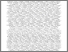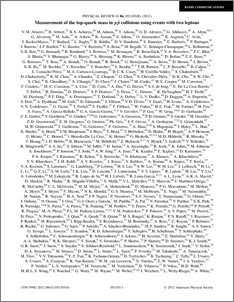# Measurement of the top quark mass in $\boldsymbol{\ppbar}$ collisions using events with two leptons

Collaboration, D0 and Bertram, Iain and Borissov, Guennadi and Fox, Harald and Ross, Anthony and Williams, Mark and Ratoff, Peter (2012) Measurement of the top quark mass in $\boldsymbol{\ppbar}$ collisions using events with two leptons. Physical Review D, 86 (5). ISSN 1550-7998Preview
PDF
PhysRevD.86.051103.pdf - Published Version

## Abstract

We present a measurement of the top quark mass ($m_t$) in $p\bar{p}$ collisions at $\sqrt{s} = 1.96$ TeV using $t\bar{t}$ events with two leptons ($ee$, $e\mu$, or $\mu\mu$) and accompanying jets in $4.3 fb^{-1}$ of data collected with the D0 detector at the Fermilab Tevatron collider. We analyze the kinematically underconstrained dilepton events by integrating over their neutrino rapidity distributions. We reduce the dominant systematic uncertainties from the calibration of jet energy using a correction obtained from $t\bar{t}$ events with a final state of a single lepton plus jets. We also correct jets in simulated events to replicate the quark flavor dependence of the jet response in data. We measure $m_t = 173.7 \pm 2.8\thinspace(\rm stat) \pm 1.5\thinspace(\rm syst)$ GeV and combining with our analysis in 1 fb$^{-1}$ of preceding data we measure $m_t = 174.0 \pm 2.4\thinspace(\rm stat) \pm 1.4\thinspace(\rm syst)$ GeV. Taking into account statistical and systematic correlations, a combination with the D0 matrix element result from both data sets yields $m_t = 173.9 \pm 1.9\thinspace(\rm stat) \pm 1.6\thinspace(\rm syst)$ GeV.

Item Type:
Journal Article
Journal or Publication Title:
Physical Review D